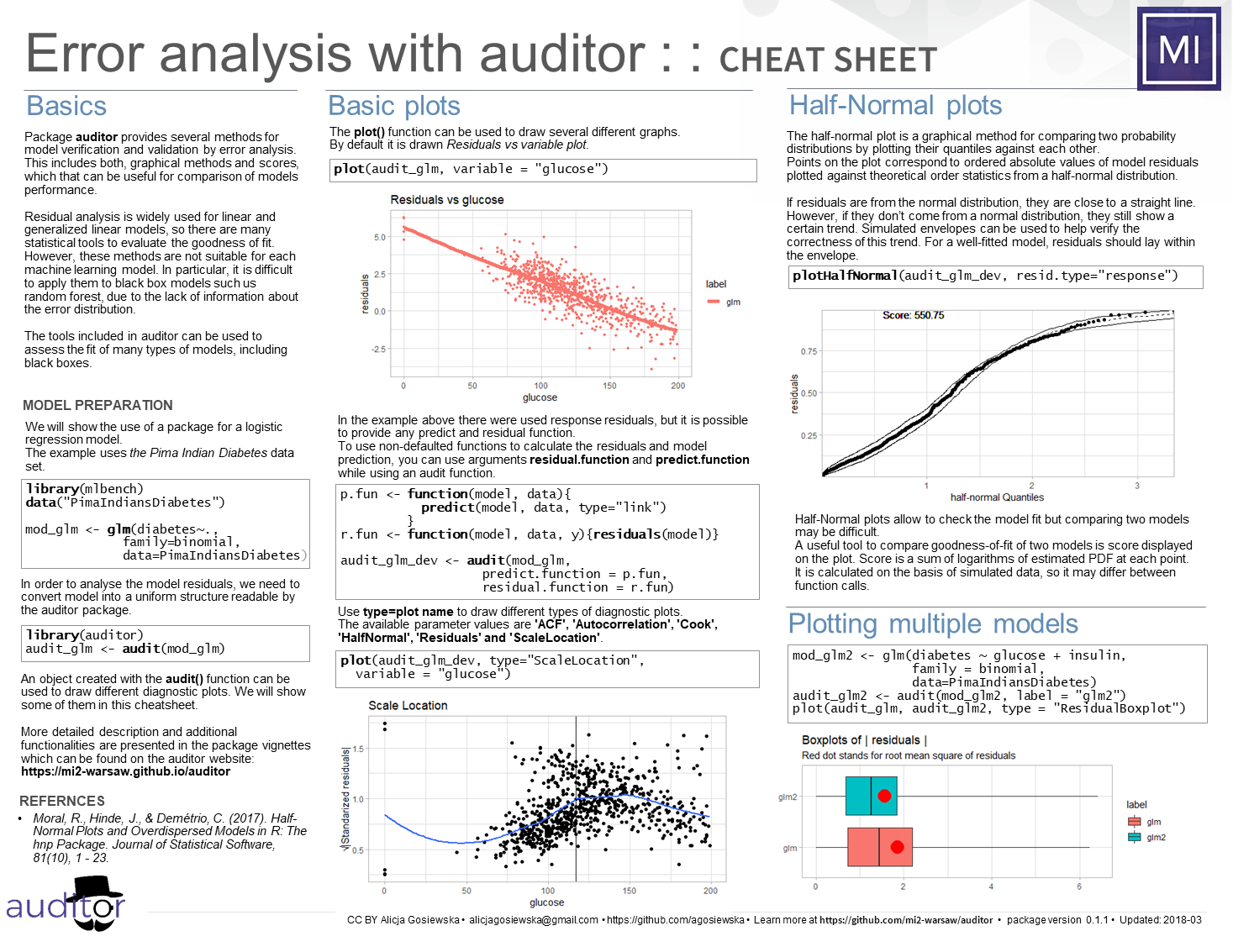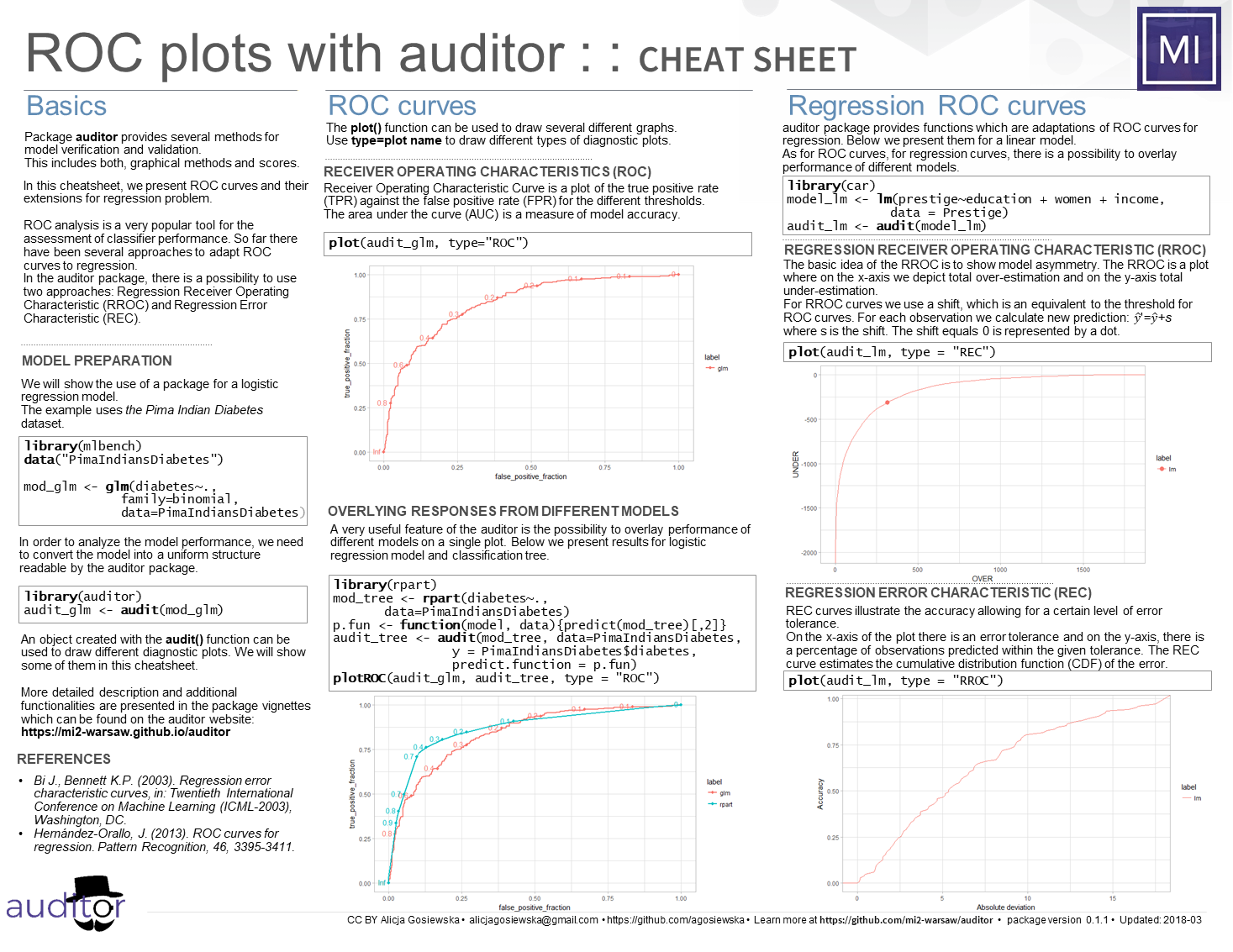# MI2DataLab/auditor

Audit of regression models
Latest commit f2095e5 Mar 11, 2019
Type Name Latest commit message Commit time
Failed to load latest commit information.Rbinder Oct 23, 2018datadocsinst Oct 3, 2018jupyter-notebooksmanmaterialstestsvignettes.Rbuildignore Aug 26, 2018.gitignore Nov 10, 2017.travis.ymlDESCRIPTIONNAMESPACENEWS.md Dec 28, 2018README.md Mar 11, 2019_pkgdown.ymlauditor.Rprojcodecov.yml

# The auditor package - model verification, validation, and error analysis## auditor's pipeline: model %>% audit() %>% plot(type=...)# Preprint

A preprint of the article about auditor is available on arxiv.

## Installation

### from GitHub

``````devtools::install_github("mi2datalab/auditor")
``````

### and from CRAN

``````install.packages("auditor")
``````

## DEMO

Run the code below or try the auditor by the online jupyter-notebook:``````library(auditor)
library(randomForest)
data(mtcars)

# fitting models
model_lm <- lm(mpg ~ ., data = mtcars)
set.seed(123)
model_rf <- randomForest(mpg ~ ., data = mtcars)

# creating a modelAudit object which contains all necessary components required for further processing
au_lm <- audit(model_lm)
au_rf <- audit(model_rf, label = "rf")

# generating plots
plot(au_lm, type = "Residual")
plot(au_lm, au_rf, type = "Residual")

plot(au_lm, au_rf, variable = "wt", type = "Prediction")

plot(au_lm, au_rf, type = "ModelCorrelation")
plot(au_lm, au_rf, values = "wt", type = "ModelCorrelation")

# plots above are availible also via plotResidual(), plotPrediction() and plotModelCorrelation() functions
``````

For more plot types and examples see A Short Overview of Plots section below.

## Cheatsheets## A Short Overview of Plots

Plot name Function Regression Classification Examples
Autocorrelation Function `plotACF()`
`plot(..., type = "ACF")`
yes yes Examples
Autocorrelation `plotAutocorrelation()`
`plot(..., type = "Autocorrelation")`
yes yes Examples
Influence of observations `plotCooksDistance()`
`plot(..., type = "CooksDistance")`
yes yes Examples
Half-Normal `plotHalfNormal()`
`plot(..., type = "HalfNormal")`
yes yes Examples
LIFT Chart `plotLIFT()`
`plot(..., type = "LIFT")`
no yes Examples
Model Correlation `plotModelCorrelation()`
`plot(..., type = "ModelCorrelation")`
yes yes Examples
Principal Component Analysis of models `plotModelPCA()`
`plot(..., type = "ModelPCA")`
yes yes Examples
Model Ranking Plot `plotModelRanking()`
`plot(..., type = "ModelRanking")`
yes yes Examples
Predicted Response vs Observed or Variable Values `plotPrediction()`
`plot(..., type = "Prediction")`
yes yes Examples
Regression Error Characteristic Curves (REC) `plotREC()`
`plot(..., type = "REC")`
yes yes Examples
Plot Residuals vs Observed, Fitted or Variable Values `plotResidual()`
`plot(..., type = "Residual")`
yes yes Examples
Residual Boxplot `plotResidualBoxplot()`
`plot(..., type = "ResidualBoxplot")`
yes yes Examples
Residual Density `plotResidualDensity()`
`plot(..., type = "ResidualDensity")`
yes yes Examples
Receiver Operating Characteristic (ROC) `plotROC()`
`plot(..., type = "ROC")`
no yes Examples
Regression Receiver Operating Characteristic (RROC) `plotRROC()`
`plot(..., type = "RROC")`
yes yes Examples
Scale-Location plot `plotScaleLocation()`
`plot(..., type = "ScaleLocation")`
yes yes Examples
Two-sided Cumulative Distribution Function `plotTwoSidedECDF()`
`plot(..., type = "TwoSidedECDF")`
yes yes Examples

## Acknowledgments

Work on this package was financially supported by the ‘NCN Opus grant 2016/21/B/ST6/02176’.

## More

Presentation during Knowledge Network Tech Meetup

You can’t perform that action at this time.